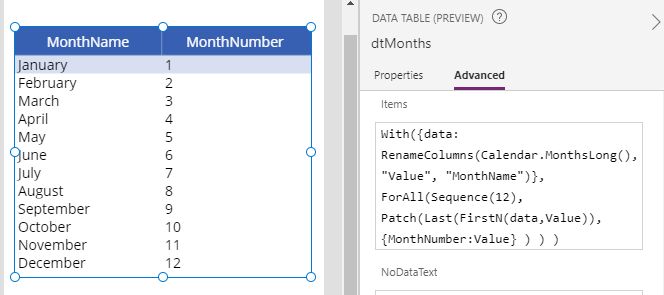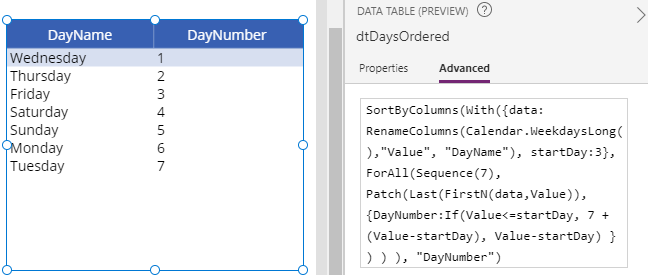# Dates - How to get a numbered list of days or months, starting from a specified day or month

For date or scheduling diary type apps, it can be very useful to retrieve a list of days or months that begin on specific day or month. This post describes some formulas that we can use.

This post highlights a set of formulas that are typically used in scheduling type applications. A common use case scenario for these types of formula is for use in drop down controls for data entry, or for specifying date elements on search screens.

The starting point for much of this logic is the calendar function. This exposes the following properties to return tables of month and weekday names in long and short format:  MonthsLong, MonthsShort, WeekdaysLong, WeekdaysShort.

## 1. Get a list of months and month numbers

To return a list of month and month numbers, we can use the following formula:

`With({data: RenameColumns(Calendar.MonthsLong(),"Value", "MonthName")},     ForAll(Sequence(12),            Patch(Last(FirstN(data,Value)),                  {MonthNumber:Value}            )    ))`This formula utilises the row numbering pattern to generate the month numbers. There are more details about this technique in my post here.

## 2. Get a list of days and day numbers

We can adapt the above formula to return day and they numbers using the following formula:

`With({data: RenameColumns(Calendar.WeekdaysLong(),"Value", "DayName")},     ForAll(Sequence(7),            Patch(Last(FirstN(data,Value)),                  {DayNumber:Value}            )    ))`

## 3.Get week days, starting from a specified day

By default, the calendar function returns Sunday as day 1. If we want to specify a different day that corresponds to day 1, we can use the formula beneath. Here, startDay defines day one. In this example, we set this to 3 in order to specify that Wednesday should be day one.

`With({data: RenameColumns(Calendar.WeekdaysLong(),"Value", "DayName"),       startDay:3},     ForAll(Sequence(7),            Patch(Last(FirstN(data,Value)),                  {DayNumber:If(Value<=startDay, 7 +(Value-startDay), Value-startDay) }            )    ))`

##Taking the source table from above, we can sort by DayNumber to return the output in ascending day number order.

`SortByColumns(    With({data: RenameColumns(Calendar.WeekdaysLong(),"Value", "DayName"),           startDay:3},         ForAll(Sequence(7),                Patch(Last(FirstN(data,Value)),                      {DayNumber:If(Value<=startDay, 7 +(Value-startDay), Value-startDay) }                )        )    ),     "DayNumber")`## 4. Get 12 months, starting from a specified month

Let's suppose that the financial year for a company starts in April. We can use the following formula to number months, with month one starting in April.

`SortByColumns(      With({data: RenameColumns(Calendar.MonthsLong(),"Value", "MonthName"),                    startMonth:4},           ForAll(Sequence(12),             Patch(Last(FirstN(data,Value)),                   {MonthNumber:If(Value<startMonth,                                   13 +(Value-startMonth),                                   Value-(startMonth-1)                                )                   }           )         )       ),       "MonthNumber")`

## Conclusion

This post described formula to retrieve a list of days or months, and how to optionally specify the day or month to begin ordering.